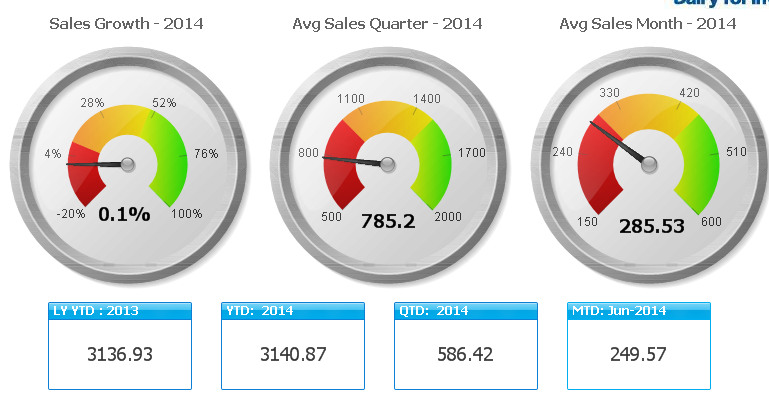# Qlik Education Discussions

Discussion Board for collaboration related to Qlik Education.

Not applicable

## Sales Growth

How to find the sales Growth in percentage?the below image is sample one.5 Replies
Not applicable

## Re: Sales Growth

You can use your formula with the metrics that you have or in which dimention you calcualte the sales growth like Year, Month, Quarter according to that use the formula

Ex:-

( sum([MT Value]) - (sum(Previos Quarter value [MT Value])))

/

sum(TOTAL  [MT Value])

In this formula it is sales growth for Quarter only.

Regards

Anand

Not applicable

## Re: Sales Growth

[MT Value]  - means Which one?

Not applicable

## Re: Sales Growth

This is example you can use your metrics

Ex:-

( sum([Your MetricValue]) - (sum(Previos Quarter value [Your MetricValue])))

/

Regards

Anand

Not applicable

## Re: Sales Growth

[Previos Quarter value] have indicate error.Which one is [Previos Quarter value]

Not applicable

## Re: Sales Growth

Hi Stefen,

Basic growth formula for sales growth is below expression but it depends on which dimension you use this expression like Year, Month and Quarter.

Suppose you want to calculate growth for current year versus previous year then

1. Fetch the current year sales value set some variables

vYTD = Year = {\$(=Max(Year))}

This will calculated by expression Sum({\$<\$(vSetYTD)> } [Sales Value])

2. Then create variable for the previous year Sum({\$<\$(vSetLYYTD)> } [Sales Value])

3. Then after all this use both expression in the gauge chart like

Ex:-

(Sum({\$<\$(vSetYTD)> } [Sales Value])

/

Sum({\$<\$(vSetLYYTD)> } [Sales Value]))

- 1

Note:- You have to use Metrics it is example i assume and fields and variables are different in your app also

Regards

Anand